## Friday, December 30, 2016

### Packing Spheres into a Cube

In this post I compare three different alignment strategies for putting spheres into a cube. Our main variable will be $$n$$, the ratio of cube side to sphere diameter. The question is, how many spheres can we get into the cube? We denote the number of spheres as $$S$$.

### Matrix Alignment

The simplest alignment of spheres to think about is a matrix alignment, or row, column, layer. If $$n=10$$, then we can fit 1000 spheres into the cube this way. Or, in general, $$S=n^3$$.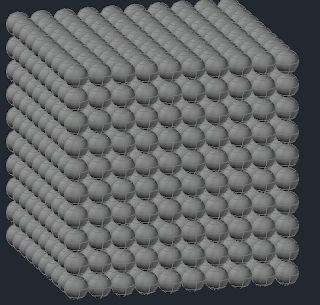Fig. 1. Matrix alignment.
One way to summarize this alignment is that each sphere belongs to its own circumscribing cube and none of these circumscribing cubes overlap each other. For small values of $$n$$, this arrangement is optimal.

### Square Base Pyramid Alignment

An improvement to $$S$$, for larger values of $$n$$ is had by making the alignment of subsequent layers offset. Start with a layer in a square arrangement ($$n$$ by $$n$$). If you look down on this first layer, it's awfully tempting to nestle the next layer of spheres in the depressions between the spheres. We will make better use of the interior space, although we won't make as good a use of the space near the edges. But if we can get enough extra layers in to make up for the losses near the outside, that would carry the day.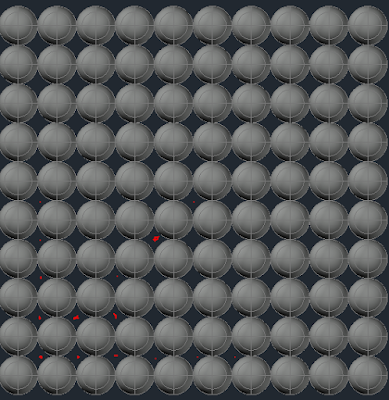Fig. 2. A few red dots show where we're thinking of putting the next layer of spheres. We won't fit as many on this layer, but the second layer isn't as far removed from the first.
In the matrix alignment, consecutive layers were all a distance of 1 unit between layers (center to center). With this current alignment, we need to know the distance between layers. We need to consider a group of four spheres as a base and another sphere nestled on top and find the vertical distance from the center of the four base spheres to the center of the top sphere. (Implicitly, the flat surface the four base spheres are resting on constitute horizontal. Vertical is perpendicular to that.)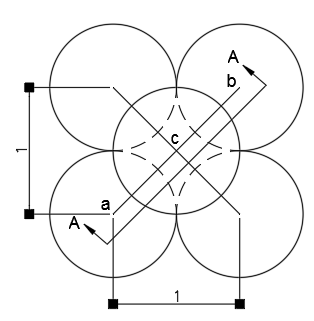Fig. 3. Top view of a square base pyramid packing arrangement.
We can see from Fig. 3., that line segment ab is  of length $$\sqrt{2}$$. We take a section parallel to this line segment, shown in Fig. 4.Fig. 4. We recognize a 45°-45°-90° triangle, abc.
We can calculate the vertical distance between layers, line segment cd, as $$\sqrt{2}/2 \approx 0.7071\dots$$. Note that the spheres in this second layer are in basically the same configuration as the bottom layer. We can see this will be the case because the top sphere in Fig. 3., has its outer circumference pass across the point where the lower spheres meet. So adjacent spheres in the second layer will be in contact. And, further, second layer spheres will follow the rectangular pattern of depressions in the bottom layer which are spaced apart the same as the centers of the spheres in the bottom layer. So we will end up with the same organization of spheres in the second layer as the bottom layer, just one fewer row and one fewer column.
We can now calculate the number of spheres that will fit in a cube using this alignment as
$$S = n^2 \lambda_1 + (n-1)^2 \lambda_2,$$
where $$\lambda_1$$ is the number of full layers and $$\lambda_2$$ is the number of "nestled" layers. (The third layer, which is indeed "nestled" into the second layer is also returned to the configuration of the first layer and so I refer to it as a full layer instead. The cycle repeats.) To find $$\lambda_1$$ and $$\lambda_2$$, we first find the total layers, $$\lambda$$ as
$$\lambda = \left \lfloor{\frac{n-1}{\sqrt{2}/2}}\right \rfloor+1$$
The rationale for this formula is: find how many spaces between layers there are and compensate for the difference between layers and spaces. Imagine a layer at the very bottom and a layer at the very top (regardless of configuration). The layer at the bottom, we get for free and is the 1 term in the formula. (The top layer may or may not be tight with the top when we're done but hold it up there for now.) The distance from the center of the bottom layer to the center of the top layer is $$n-1$$. The number of times we can fit our spacing interval into this number (completely), is the number of additional layers we can fit in. (Additional to the bottom layer, the top layer is the last of the layers we just found room for in the $$n-1$$, or, technically, $$n-1/2$$, er, whatever, do some examples.)
The break down between $$\lambda_1$$ and $$\lambda_2$$ will not be exactly even, with $$\lambda_1$$ having one more if $$\lambda$$ is odd. We can show this neatly using the ceiling operator
$$\lambda_1 = \left \lceil{\lambda/2}\right \rceil$$
$$\lambda_2 = \lambda - \lambda_1$$
As early as $$n=4$$ we get $$S=66$$, which is better than the matrix arrangement by 2 spheres. I modeled this to make sure I didn't let an off by one error sneak into my formula (see Fig. 5.).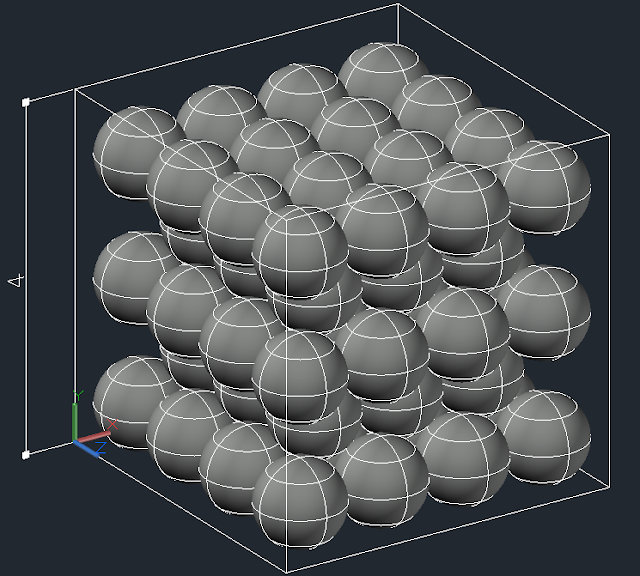Fig. 5. Somewhat surprisingly, rearrangement of spheres (relative to matrix alignment) produces gains in volume coverage even at $$n=4$$.
Here it is in Maxima:

### Tetrahedral Alignment

Another rearrangement is similar to a honeycomb. A honeycomb is an arrangement of hexagons that meet perfectly at their edges. Pictures of this are readily available online. This Wikipedia article suffices. Imagine putting a circle or a sphere in each of these honeycomb cells. The will produce a single layer which is more compact, although it will push the distance between layers apart.

So, what do we need to know to make a formula for this one?
1. Find the distance between successive layers.
2. Formula for the number of layers.
3. Formula for the number of spheres in each layer.
1. This depends on knowing the range of layer configurations (we will only have two different layer configurations).
4. Some assembly required.
This is the same procedure we followed already for the square based pyramid alignment, but we are being more explicit about the steps.Fig. 6. We need to find the distance of line segment ad.
We recognize we have an equilateral triangle and so we can identify a 30°-60°-90° triangle on each half of altitude ab. We have drawn all of the angle bisectors for this triangle which meet at a common point (commonly known property for triangles). We can recognize/calculate the length of bd as $$\frac{1}{2\sqrt{3}}$$. We can then calculate the length of ad as $$\frac{\sqrt{3}}{2} - \frac{1}{2\sqrt{3}} = 1/\sqrt{3}$$. From the Pythagorean theorem, we have a length for cd of $$\sqrt{2/3}$$.
The number of spheres in the first layer will require us to use the distance for ab. We now need to find the number of rows in the bottom layer. We have
$$L_1=n \rho_1 + (n-1) \rho_2,$$
where $$\rho_1$$ and $$\rho_2$$ are the number of each kind of row in the layer and $$L_1$$ is the number of spheres in the first layer. Since rows are spaced by $$\sqrt{3}/2$$, we have a number of rows, $$\rho$$, of
$$\rho = \left \lfloor{\frac{n-1}{\sqrt{3}/2}}\right \rfloor+1$$
and $$\rho_1$$ and $$\rho_2$$ can be broken out similar to our $$\lambda$$'s earlier.
$$\rho_1 = \left \lceil{\frac{\rho}{2}}\right \rceil$$
$$\rho_2 = \rho - \rho_1.$$Fig. 7. We're looking for the distance of line segment cd which can be found using ac and ad.
Now, there is a little matter of how nicely (or not) the second layer will behave for us. It is noteworthy that we are not able to put a sphere into every depression. We skip entire rows of depressions. (The Wikipedia article on sphere packing referred to these locations as the "sixth sphere".) These skipped locations may play mind games on you as you imagine going to the third layer. Nevertheless, the third layer can be made identical to the first, which is what I do here.Fig. 8. The first layer is blue. The second layer is red. Are we going to have trouble with you Mr. Hedral?
There's a couple of things that happen on the second layer. First, instead of starting with a row of size $$n$$, we start with a row of size $$n-1$$. The second issue is that we may not get as many total rows in. We could do the same formula for rows again but now accounting for the fact that we have lost an additional $$\frac{1}{2\sqrt{3}}$$ before we start. However, we can reduce this to a question of "do we lose a row or not?" The total distance covered by the rows, $$R$$, for the bottom layer is
$$R = (\rho-1) \frac{\sqrt{3}}{2} + 1$$
If $$n-R\ge\frac{1}{2\sqrt{3}}$$, then we have the same number of total rows. Otherwise, we have one less row. We let $$r_L$$ represent the number of rows lost in the second layer, which will either be 0 or 1. Noting that the order of the rows is now $$n-1$$ followed by $$n$$, we can give an expression for the number of spheres, $$L_2$$, in our second layer now.
$$L_2 = (n-1)(\rho_1 - r_L) + n\rho_2$$
We have a very similar formula for the total number of spheres in the box.
$$S = L_1\lambda_1 + L_2\lambda_2,$$
where
$$\lambda_1 = \left \lceil{\frac{\lambda}{2}}\right \rceil$$
$$\lambda_2 = \lambda - \lambda_1,$$
where
$$\lambda = \left \lfloor{\frac{n-1}{\sqrt{2/3}}}\right \rfloor+1.$$
My Maxima function for this is

### Comparison Between Methods

It isn't too hard to tell that one of the two second approaches is going to produce better results than the first after some value of $$n$$ (Fig. 9.). What is more surprising is that our latter two alignments stay neck and neck up into very high values of $$n$$. If we divide them by the volume of the containing cube, both appear to be a round about way to arrive at the square root of 2! (Fig. 10.)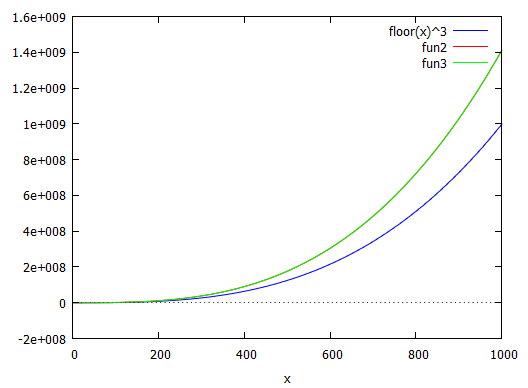Fig. 9. Both of the latter approaches give much better packing densities than the matrix alignment.Fig. 10. Square base packing in blue, tetrahedral packing in red, both divided by total volume (# of spheres/unit volume). Unclear if there is a distinct winner and we seem to be getting closer to $$\sqrt{2}$$.

Let's see which one wins more often for positive integer values of $$n$$ in Maxima.

So, there's no run away winner, but if you're a betting man, bet on square base pyramid packing out of the available choices in this post. Regardless, it appears that both of these packing arrangements approach optimal packing (see Sphere packing). My density calculation (allowing the $$\sqrt{2}$$ conjecture) for volume coverage comes out to
Gauss, old boy, seems to have approved of this number.